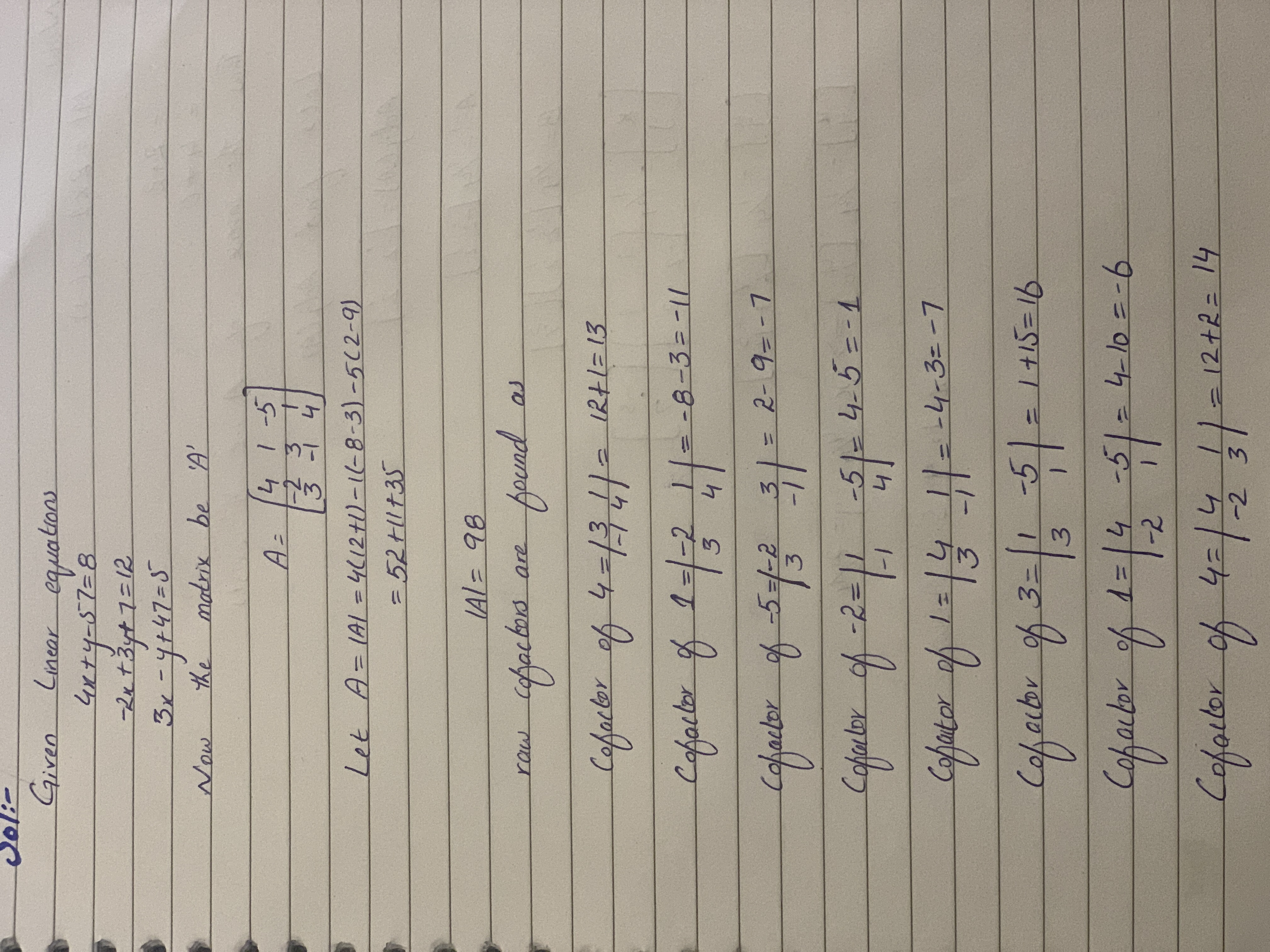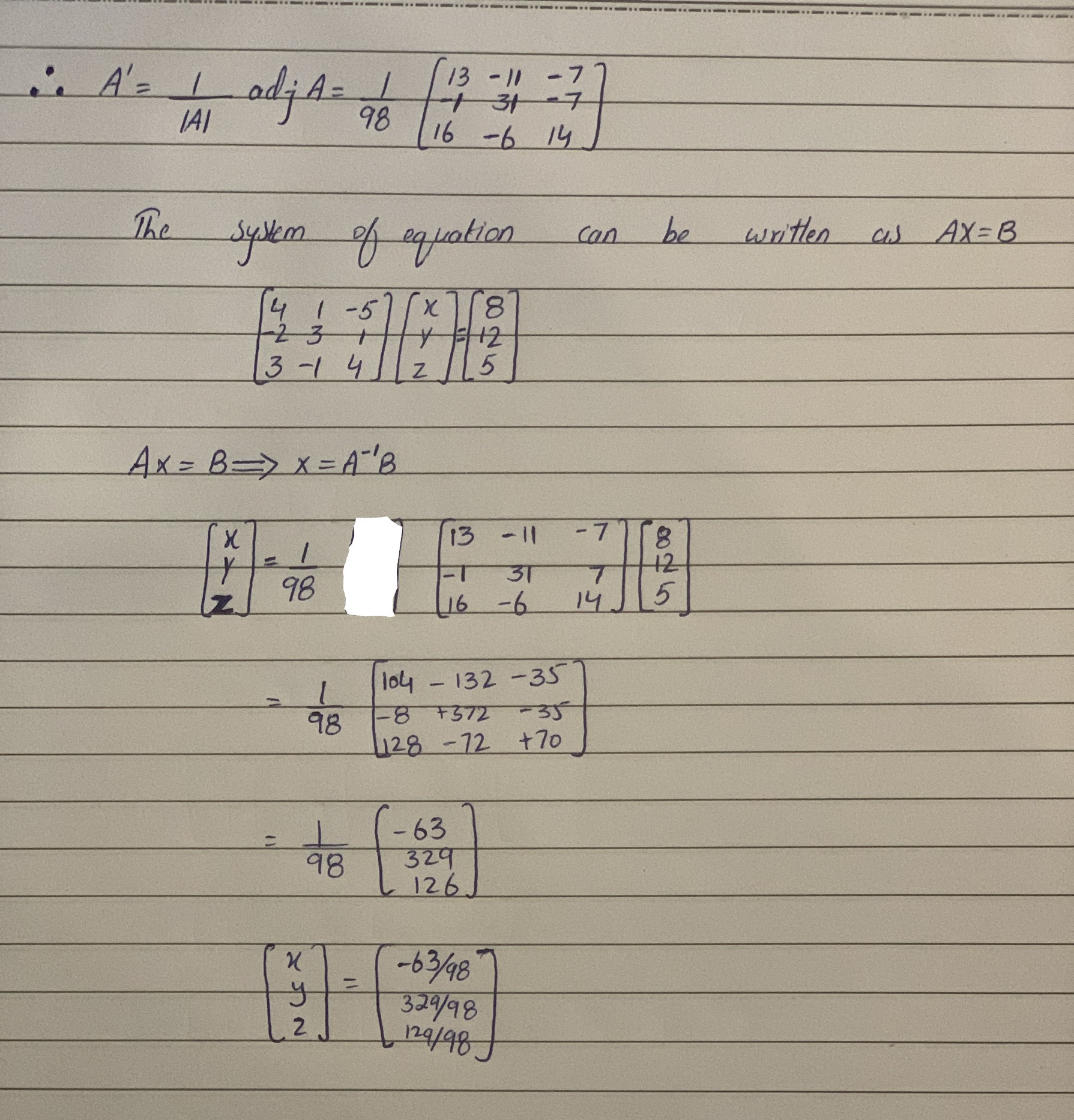# For the given a system of linear equations 4x+y-5z=8 -2x+3y+z=12 3x-y+4z=5 Use matrix inversion to solve simultaneous equations.Tammy Todd 2021-02-19 Answered
For the given a system of linear equations
4x+y-5z=8
-2x+3y+z=12
3x-y+4z=5
Use matrix inversion to solve simultaneous equations.
You can still ask an expert for help

• Live experts 24/7
• Questions are typically answered in as fast as 30 minutes
• Personalized clear answers

Solve your problem for the price of one coffee

• Math expert for every subject
• Pay only if we can solve itFatema Sutton
This question is solved as follows:We have step-by-step solutions for your answer!Jeffrey Jordon

Given Linear equations

$4x+y-5z=8$

$-2x+3y+z=12$

$3x-y+4z=5$

Now the matrix be 'A'

$A=\left[\begin{array}{ccc}4& 1& -5\\ -2& 3& 1\\ 3& -1& 4\end{array}\right]$

Let $A=|A|=4\left(12+1\right)-1\left(-8-3\right)-5\left(2-9\right)$

$=52+11+35$

$|A|=98$

raw cofactors are found as

Cofactor of $4=\left[\begin{array}{cc}3& 1\\ -1& 4\end{array}\right]=12+1=13$

Cofactor of $1=\left[\begin{array}{cc}-2& 1\\ 3& 4\end{array}\right]=-8-3=-11$

Cofactor of $-5=\left[\begin{array}{cc}-2& 3\\ 3& -1\end{array}\right]=2-9=-7$

Cofactor of $-2=\left[\begin{array}{cc}1& -5\\ -1& 4\end{array}\right]=4-5=-1$

Cofactor of $1=\left[\begin{array}{cc}4& 1\\ 3& -1\end{array}\right]=-4-3=-7$

Cofactor of $3=\left[\begin{array}{cc}1& -5\\ 3& 1\end{array}\right]=1+15=16$

Cofactor of $1=\left[\begin{array}{cc}4& -5\\ -2& 1\end{array}\right]=4-10=-6$

Cofactor of $4=\left[\begin{array}{cc}4& 1\\ -2& 3\end{array}\right]=12+2=14$

$\therefore {A}^{\prime }=\frac{1}{|A|}adjA=\frac{1}{98}\left[\begin{array}{ccc}13& -11& -7\\ -1& 31& -7\\ 16& -6& 14\end{array}\right]$

The system of equation can be written as AX=B

$\left[\begin{array}{ccc}4& 1& -5\\ -2& 3& 1\\ 3& -1& 4\end{array}\right]\left[\begin{array}{c}x\\ y\\ z\end{array}\right]=\left[\begin{array}{c}8\\ 12\\ 5\end{array}\right]$

$Ax=B⇒x={A}^{-1}B$

$\left[\begin{array}{c}x\\ y\\ z\end{array}\right]=\frac{1}{98}\left[\begin{array}{ccc}13& -11& -7\\ -1& 31& 7\\ 16& -6& 14\end{array}\right]\left[\begin{array}{c}8\\ 12\\ 5\end{array}\right]$

$=\frac{1}{98}\left[\begin{array}{ccc}104& -132& -35\\ -8& +372& -35\\ 128& -72& 70\end{array}\right]$

$=\frac{1}{98}\left[\begin{array}{c}-63\\ 329\\ 126\end{array}\right]$

$\left[\begin{array}{c}x\\ y\\ z\end{array}\right]=\left[\begin{array}{c}-63/98\\ 329/98\\ 129/98\end{array}\right]$

We have step-by-step solutions for your answer!